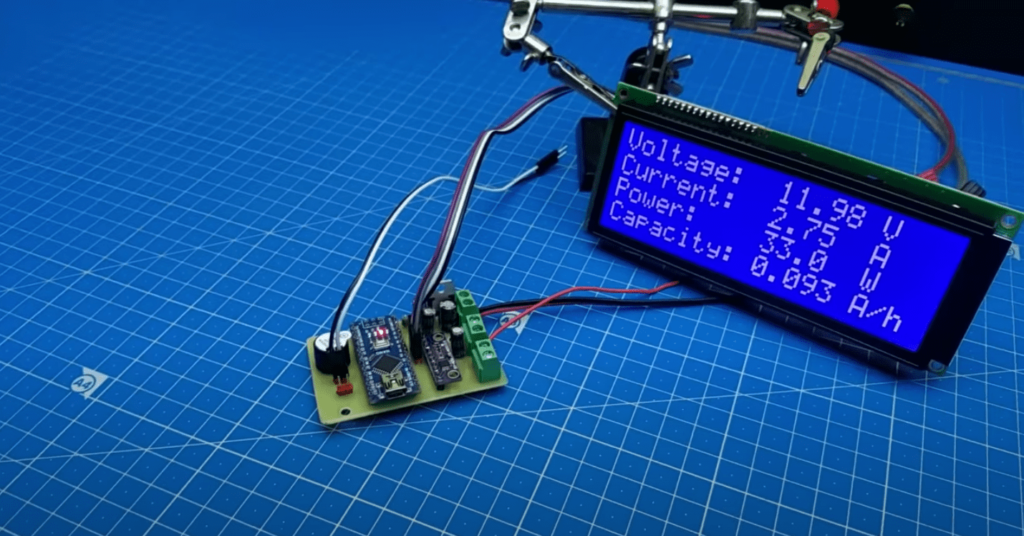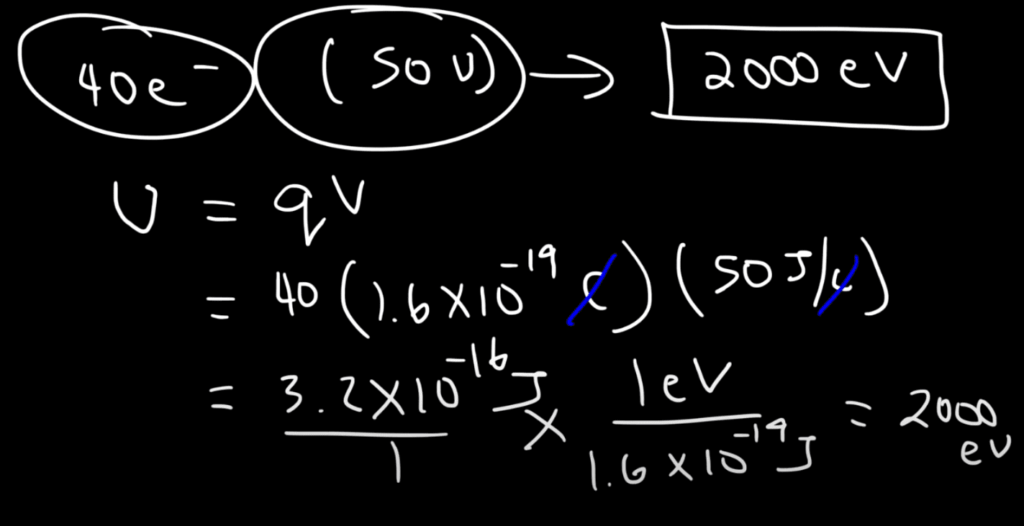# Volt to Electron-Volt Conversion Calculator

Welcome to the Volt to Electron-Volt (V to eV) Calculator! This online calculator allows you to easily convert voltage in volts (V) to electron-volts (eV) using the formula eV = V × e. To use this calculator, simply input the definition of a volt and the definition of elementary charge, and click “Calculate” to get the result in electron volts. This tool is useful for students, researchers, and anyone who works with voltage and electron volts in various fields such as physics, chemistry, and engineering. Try it out now and simplify your voltage conversion calculations!

Contents

## Why May You Need Volt to Electron-Volt Calculator?

There are many reasons why you may need a Volt to Electron-Volt Calculator. Here are a few examples:

• Education: If you are a student studying physics, chemistry, or engineering, you may need to convert between volts and electron volts for your coursework or experiments.
• Research: If you are a researcher working in a field that involves voltage, such as materials science or electronics, you may need to convert between volts and electron volts to analyze and interpret your data.
• Industry: If you work in an industry that involves voltage, such as electrical engineering or semiconductor manufacturing, you may need to convert between volts and electron volts for various calculations and measurements.
• Personal interest: If you have a personal interest in physics or electronics, you may want to use a Volt to Electron-Volt Calculator to understand better the concepts and applications of voltage and electron volts.

Overall, a Volt to Electron-Volt Calculator can be a helpful tool for anyone who needs to convert between volts and electron volts in their work, studies, or personal interests.

## How to Convert Volts to Electron-Volts?

To convert volts (V) to electron volts (eV), you can use the following formula:

eV = V × e

where “e” is the elementary charge, which is approximately equal to 1.602 x 10^-19 coulombs.

Here’s an example of how to use this formula:

Let’s say you have a voltage of 10 volts. To convert this to electron volts, you would use the formula:

eV = 10 V × 1.602 x 10^-19 C
eV = 1.602 x 10^-18 eVTherefore, 10 volts is equal to 1.602 x 10^-18 electron-volts.

## How to Calculate Electron-Volts From Coulombs?

To calculate electron-volts (eV) from Coulombs (C), you need to know the voltage (V) across the electric potential difference. You can use the following formula:

eV = V × C / e

where “e” is the elementary charge, which is approximately equal to 1.602 x 10^-19 coulombs.

Here’s an example of how to use this formula:

Let’s say you have a charge of 1 microcoulomb (1 x 10^-6 C) and a voltage of 100 volts. To calculate the electron-volts, you would use the formula:

eV = 100 V × 1 x 10^-6 C / 1.602 x 10^-19 C
eV = 6.241 x 10^14 eV

Therefore, the charge of 1 microcoulomb across a voltage of 100 volts is equal to 6.241 x 10^14 electron-volts.

## FAQ

### How many volts is 1 electron-volt?

One electron-volt (eV) is not equal to any specific number of volts since volts and electron-volts are units of different physical quantities.

Electron-volt is a unit of energy equal to the amount of energy gained or lost by an electron when it moves through an electric potential difference of one volt. In contrast, a volt is a unit of electric potential difference, representing the energy required to move one coulomb of charge through an electric field.

However, you can use the formula eV = V × e to calculate the voltage corresponding to a given amount of energy in electron-volts, provided you know the elementary charge “e”. The elementary charge is approximately equal to 1.602 x 10^-19 coulombs.

### How many electrons are in 1 volt?

The number of electrons in 1 volt (V) depends on the context of the question. A volt is a unit of electric potential difference, representing the energy required to move one coulomb of charge through an electric field. Therefore, the number of electrons in 1 volt depends on the amount of charge being moved.

For example, if you have a charge of 1 coulomb (C), then moving this charge across an electric potential difference of 1 volt would involve moving a certain number of electrons. One coulomb of charge is equal to approximately 6.24 x 10^18 electrons, so moving 1 coulomb of charge across 1 volt would involve moving approximately 6.24 x 10^18 electrons.

However, if you have a different amount of charge or a different electric potential difference, the number of electrons involved would be different.

### What is meant by 1 eV?

In physics, 1 eV (electron-volt) is a unit of energy that represents the amount of energy gained or lost by a single electron when it moves through an electric potential difference of one volt.

More specifically, an electron-volt is defined as the amount of energy that an electron acquires when it moves across an electric potential difference of one volt in a vacuum. It is equal to approximately 1.602 x 10^-19 joules.

The electron-volt is a commonly used unit of energy in atomic and subatomic physics, where the energies involved are often very small. It is particularly useful for describing the energy levels of electrons in atoms and the energies of subatomic particles in particle accelerators.

## Related Video: Electron-Volt Explained, Conversion to Joules, Basic Introduction

The Volt to Electron-Volt Calculator is a helpful tool that easily converts between volts and electron-volts using the formula eV = V x e. Whether you are studying physics or need to make quick conversions between these two units of measurement, this calculator can help you save time and avoid errors. We hope that you find this tool helpful and easy to use.

Scroll to Top